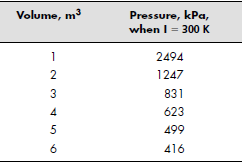# Lesson 12: Numerical Techniques

## Objectives

• To interpolate between data points, using either linear or cubic spline models.
• To solve differential equations numerically.

## Questions

Consider a gas in a piston–cylinder device in which the temperature is held constant. As the volume of the device was changed, the pressure was measured. The volume and pressure values are reported in the following table:1. Use linear interpolation to estimate the pressure when the volume is 3.8 m3.
2. Use cubic spline interpolation to estimate the pressure when the volume is 3.8 m3.
3. Use linear interpolation to estimate the volume if the pressure is measured to be 1000 kPa.
4. Use cubic spline interpolation to estimate the volume if the pressure is measured to be 1000 kPa.
2. Use the interp2 function and the data to approximate a pressure value when the volume is 5.2 m3 and the temperature is 425 K.
3. Fit the data with first-, second-, third-, and fourth-order polynomials, using the polyfit function:
• Plot your results on the same graph.
• Plot the actual data as a circle with no line.
• Calculate the values to plot from your polynomial regression results at intervals of 0.2 m3.
• Do not show the calculated values on the plot, but do connect the points with solid lines.
• Which model seems to do the best job? (Hit: compare the values of the sum-of-the squares calculation of each model.)
4. Using a polynomial to model a function can be very useful, but it is always dangerous to extrapolate beyond your data. We can demonstrate this pitfall by modeling a sine wave as a third-order polynomial.
1. Define x = -1:0.1:1
2. Calculate y = sin(x)
3. Use the polyfit function to determine the coefficients of a third-order polynomial to model these data.
4. Use the polyval function to calculate new values of y (modeled_y) based on your polynomial, for your x vector from -1 to 1.
5. Plot both sets of values on the same graph. How good is the fit?
6. Create a new x vector, new_x = -4:0.1:4.
7. Calculate new_y values by finding sin(new_x).
8. Extrapolate new_modeled_y values by using polyval with the coefficient vector you found in part (c), and the new_x values.
9. Plot both new sets of values on the same graph. How good is the fit outside of the region from -1 to 1?